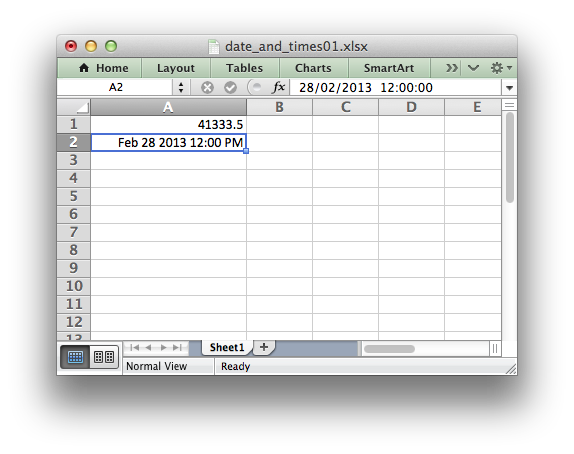libxlsxwriter
dates_and_times01.c

Example of writing a dates and time in Excel using a number with date formatting. This demonstrates that dates and times in Excel are just formatted real numbers. An easier approach using a lxw_datetime struct is shown in the next example./*
* Example of writing a dates and time in Excel using a number with date
* formatting. This demonstrates that dates and times in Excel are just
* formatted real numbers.
*
* An easier approach using a lxw_datetime struct is shown in example
* dates_and_times02.c.
*
* Copyright 2014-2021, John McNamara, jmcnamara@cpan.org
*
*/
#include "xlsxwriter.h"
int main() {
/* A number to display as a date. */
double number = 41333.5;
/* Create a new workbook and add a worksheet. */
lxw_workbook *workbook = workbook_new("date_and_times01.xlsx");
lxw_worksheet *worksheet = workbook_add_worksheet(workbook, NULL);
/* Add a format with date formatting. */
lxw_format *format = workbook_add_format(workbook);
format_set_num_format(format, "mmm d yyyy hh:mm AM/PM");
/* Widen the first column to make the text clearer. */
worksheet_set_column(worksheet, 0, 0, 20, NULL);
/* Write the number without formatting. */
worksheet_write_number(worksheet, 0, 0, number, NULL ); // 41333.5
/* Write the number with formatting. Note: the worksheet_write_datetime()
* or worksheet_write_unixtime() functions are preferable for writing
* dates and times. This is for demonstration purposes only.
*/
worksheet_write_number(worksheet, 1, 0, number, format); // Feb 28 2013 12:00 PM
return workbook_close(workbook);
}
workbook_close
lxw_error workbook_close(lxw_workbook *workbook)
Close the Workbook object and write the XLSX file.
workbook_new
lxw_workbook * workbook_new(const char *filename)
Create a new workbook object.
format_set_num_format
void format_set_num_format(lxw_format *format, const char *num_format)
Set the number format for a cell.
lxw_worksheet
Struct to represent an Excel worksheet.
Definition: worksheet.h:2107
lxw_format
Struct to represent the formatting properties of an Excel format.
Definition: format.h:358
lxw_workbook
Struct to represent an Excel workbook.
Definition: workbook.h:280
worksheet_write_number
lxw_error worksheet_write_number(lxw_worksheet *worksheet, lxw_row_t row, lxw_col_t col, double number, lxw_format *format)
Write a number to a worksheet cell.
worksheet_set_column
lxw_error worksheet_set_column(lxw_worksheet *worksheet, lxw_col_t first_col, lxw_col_t last_col, double width, lxw_format *format)
Set the properties for one or more columns of cells.